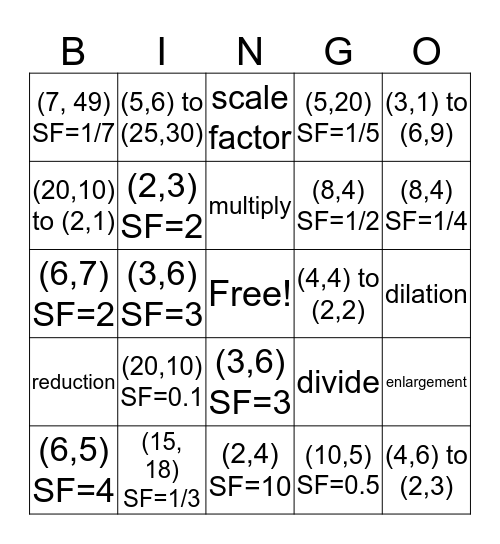# DilationThis bingo card has a free space and 24 words: (20,10) SF=0.1, (15, 18) SF=1/3, multiply, (6,5) SF=4, (3,1) to (6,9), (7, 49) SF=1/7, (20,10) to (2,1), (5,6) to (25,30), (4,4) to (2,2), (3,6) SF=3, (8,4) SF=1/2, (6,7) SF=2, divide, enlargement, (2,3) SF=2, (10,5) SF=0.5, dilation, scale factor, (8,4) SF=1/4, (3,6) SF=3, (4,6) to (2,3), reduction, (5,20) SF=1/5 and (2,4) SF=10.

⚠ This card has duplicate items: (3,6) SF=3 (2)

## Play Online